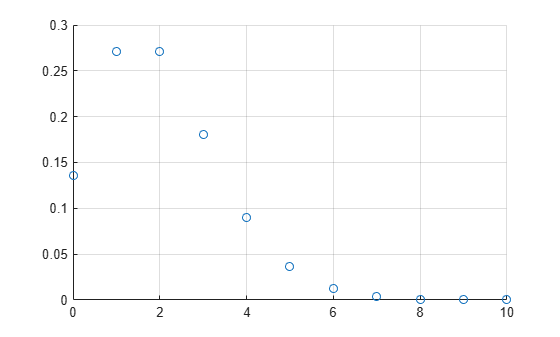# poisspdf

Poisson probability density function

## Syntax

``y = poisspdf(x,lambda)``

## Description

example

````y = poisspdf(x,lambda)` computes the Poisson probability density function at each of the values in `x` using the rate parameters in `lambda`.`x` and `lambda` can be scalars, vectors, matrices, or multidimensional arrays that all have the same size. If only one argument is a scalar, `poisspdf` expands it to a constant array with the same dimensions as the other argument.```

## Examples

collapse all

Compute and plot the Poisson probability density function for the specified range of integer values and average rate.

In the computer hard disk manufacturing process, flaws occur randomly. Assuming that on average a 4 GB hard disk has two flaws, compute the probability that a disk has no flaws.

`poisspdf(0,2)`
```ans = 0.1353 ```

Compute the Poisson probability density function values at each value from 0 to 10. These values correspond to the probabilities that a disk has 0, 1, 2, ..., 10 flaws.

```flaws = 0:10; y = poisspdf(flaws,2);```

Plot the resulting probability values.

```scatter(flaws,y,'Marker',"o") grid on```## Input Arguments

collapse all

Values at which to evaluate the Poisson pdf, specified as a scalar value or array of scalar values. For noninteger values `x`, the Poisson probability density function is zero.

Example: `[0,1,3,4]`

Data Types: `single` | `double`

Rate parameters, specified as a positive value or array of positive values. The rate parameter indicates the average number of events in a given time interval.

Example: `2`

Data Types: `single` | `double`

## Output Arguments

collapse all

Poisson pdf values, returned as a scalar value or array of scalar values. Each element in `y` is the Poisson pdf value of the distribution evaluated at the corresponding element in `x`.

Data Types: `single` | `double`

collapse all

### Poisson Probability Density Function

The Poisson probability density function lets you obtain the probability of an event occurring within a given time or space interval exactly x times if on average the event occurs λ times within that interval.

The Poisson probability density function for the given values x and λ is

`$f\left(x|\lambda \right)=\frac{{\lambda }^{x}}{x!}{e}^{-\lambda }\text{\hspace{0.17em}};\text{\hspace{0.17em}}x=0,1,2,\dots ,\infty \text{\hspace{0.17em}}.$`

## Alternative Functionality

• `poisspdf` is a function specific to Poisson distribution. Statistics and Machine Learning Toolbox™ also offers the generic function `pdf`, which supports various probability distributions. To use `pdf`, specify the probability distribution name and its parameters. Alternatively, create a `PoissonDistribution` probability distribution object and pass the object as an input argument. Note that the distribution-specific function `poisspdf` is faster than the generic function `pdf`.

• Use the Probability Distribution Function app to create an interactive plot of the cumulative distribution function (cdf) or probability density function (pdf) for a probability distribution.

## Version History

Introduced before R2006a